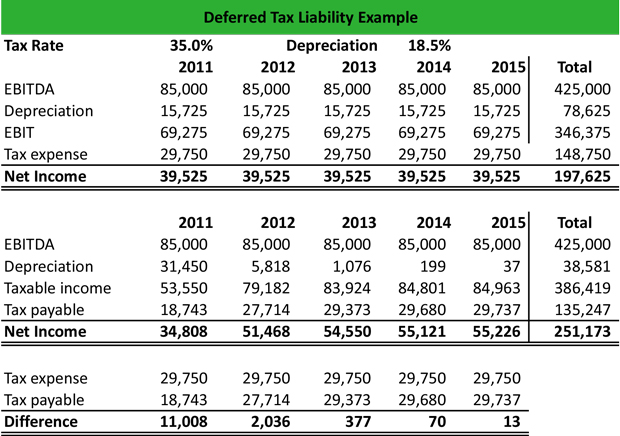# What is a Deferred Tax Liability (DTL)?

Definition: Deferred tax liability (DTL) is an income tax obligation arising from a temporary difference between book expenses and tax deductions that is recorded on the balance sheet and will be paid in a future accounting period.

## What Does Deferred Tax Liability Mean?

What is the definition of deferred tax liability? DTL is reported on a firm’s balance sheet and represents the net difference between the taxes that are paid in the current accounting period and the taxes that will be paid in the next accounting period. The liability occurs when the accounting income is greater than the taxable income.

In this case, the company is allowed to defer taxes on a percentage of its income and report this amount as a DTL on its balance sheet. When the tax is due, there will be an equal amount reduction in the DTL item and the cash and cash equivalents account on the balance sheet.

Let’s look at an example.

## Example

Jonathan is an accountant in a retail company. He is asked to calculate the deferred taxes for the period 2011 – 2015, and see if there are deferred tax liabilities or deferred tax assets, or both.

Jonathan collects all relevant information from the company’s balance sheet and income statement and creates the following Excel spreadsheet:Based on a tax rate of 35% and a depreciation of 18.5%, Jonathan calculates the net income for reporting purposes, which is \$197,625 and the net income for accounting purposes which is \$251,173.

Note that in reporting purposes table, the depreciation is reported as 18.5% every year, whereas in the accounting purposes table, the depreciation is calculated as \$15,725 x 2 = \$31,450 in 2011. Based on this amount, Jonathan applies the 18.5%.

In the end, the difference is calculated based on the tax expense that is reported minus the calculated tax payable. The positive difference indicated that there is a DTL.

## Summary Definition

Define Deferred Tax Liabilities: DTL means an accrued tax on the books because book expenses did not match tax deductions for a particular year.